blog

# Bear Put Spread Payoff Diagram: A Beginners Guide

#### Options Trading 101 - The Ultimate Beginners Guide To Options

As Seen On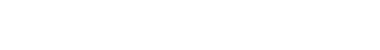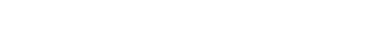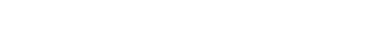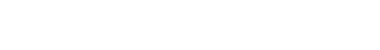by Gavin in Blog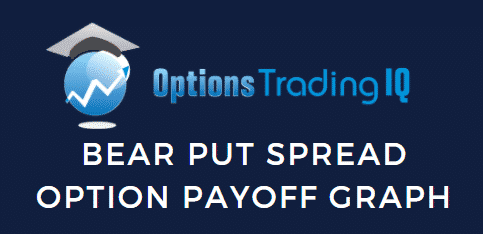Today we’re going to look at the bear put spread payoff diagram. This strategy is also sometimes called a bear debit spread or put debit spread.

This is designed for beginners so they can develop a basic understanding how to read the payoff graphs.

We’ll look at:

## Bear Put Spread Option Basics

A bear put spread is one of the easiest option trades and a great place for beginners to start their learning journey.

A bear put spread option is 2 contracts between a buyer and seller. It is an options strategy that is used when the investor expects a moderate drop in the price of the underlying asset. The strategy uses two put options to form a range consisting of a high strike price and a low strike price

## Bear Put Spread Payoff Diagram

Understanding payoff graphs (or diagrams as they are sometimes referred) is absolutely essential for option traders.

A payoff graph will show the option position’s total profit or loss (Y-axis) depending on the underlying price (x-axis).

Here is an example: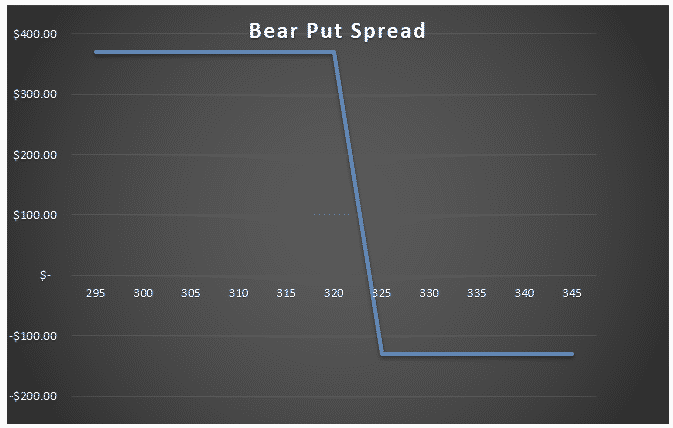What we are looking at here is the payoff graph for a bear put spread option strategy.

In this example the trader has bought a \$325 strike put and sold a \$320 strike put for a net \$1.30 per contract (or \$130 for a standard option contract representing 100 shares).

The premium paid of \$130 is the most the trade can lose. That occurs at an underlying stock price of \$325 and above.

Below the strike price of \$325, the increases as the stock price drops.

Below \$320 is where the max profit is at expiration.

The lines crosses into profit at a certain point when the stock price fall below the breakeven point.

The breakeven point is simply the long strike price minus the premium paid. In this case it would be: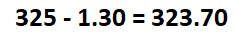If the premium paid had been slightly higher at \$2, then the breakeven price would be \$323

## Different Scenarios At Expiration

Four different scenarios can occur at expiration for a bear put spread trade.

### 1. Underlying stock price is below the short strike price

This is the ideal scenario that the trader was hoping for. If the underlying stock price is below the short strike price at expiration, the bear put spread will be in-the-money, the long put will be exercised at \$325 and short put assigned at \$320.

The profit for the trade will be equal to the width of the strikes minus premium paid.

In our example, this would be \$5 – \$1.30 = \$3.70 per contract or \$370 in total.

The trader can either let the options exercised and assigned automatically by the broker or sell them back in the market for a profit.

### 2. Underlying stock price is above the short strike price but below the breakeven price

If the stock is above the short strike price at expiration, the trader may think the trade has a loss, but unless it gets above the breakeven price, it will not end in a loss.

For example, let’s say the stock ends at \$322. The \$325 strike long put option has \$3 of profit and it will be exercised. The \$320 strike short put option will expire worthless.

The \$3 of profit is more than the \$1.30 paid. So in this scenario, the trader has profit \$1.70 per contract.

### 3. Underlying stock price is above the breakeven price but below the long strike price

If the stock is above the breakeven price at expiration, the trader may think the trade has a max loss, but unless it gets above the long strike price, it will not end in a max loss.

For example, let’s say the stock ends at \$324. The \$325 strike long put option has \$1 of profit and it will be exercised. The \$320 strike short put option will expire worthless.

The \$1 of profit minus \$1.30 premium paid is less than the max loss of \$1.30 premium paid.

So in this scenario, the trader has loss of only \$0.30 per contract compared to max loss of \$1.30.

### 4. Underlying stock price is above the long put price

If the underlying stock price is above the long strike price at expiration, the bear put spread option will expire worthless for both strikes. The loss for the trade will be equal to the premium paid.

In our example, this would be \$1.30 per contract or \$130 in total.

The good thing about a bear put spread is that the loss is limited to the premium paid, so it doesn’t matter how high the stock goes, the most the trader can lose is \$130 in this example.

## Payoff Graph At Interim Dates

So far, we’ve only considered the payoff graph at expiration. The payoff graph at interim dates will look very different and will be impacted by factors such as time decay and implied volatility.

Calculating the profit and loss at expiration is quite simply, using excel or even in your head if you are half decent at maths.

Calculating profits and losses at interim dates is much more complex and requires more advanced software than just Excel.

Any decent options broker will have the ability to show option profits and losses at interim dates.

The below bear put spread option payoff is from Interactive Brokers.

The bear put spread option was an AAPL 100/95 strike put spread bought at \$1.30 per contract or \$130 in total. The breakeven price at expiration is \$98.70 (long strike price minus the premium paid).

The blue line shows the expiration payoff that you are now familiar with and the purple line shows what is known as a “T+0” line.

T+0 simply means, what does the trade look like as of today?

Notice that the breakeven price as of today is the current AAPL price. This makes sense because we just bought the bear put spread so wouldn’t expect to have made or lost any money yet.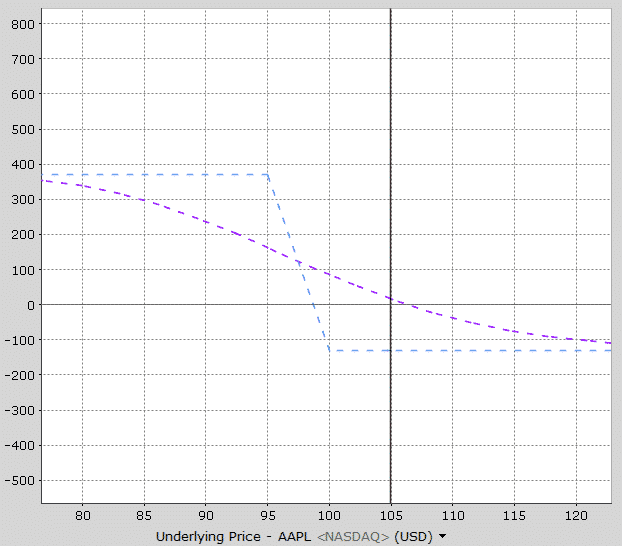Notice also that profits occur before the expiration breakeven price and below 95, the profits are not the maximum profit.

As time goes by, the interim line slowly moves towards the expiration line.

Here’s how the payoff diagram is estimated to look at one week before expiration.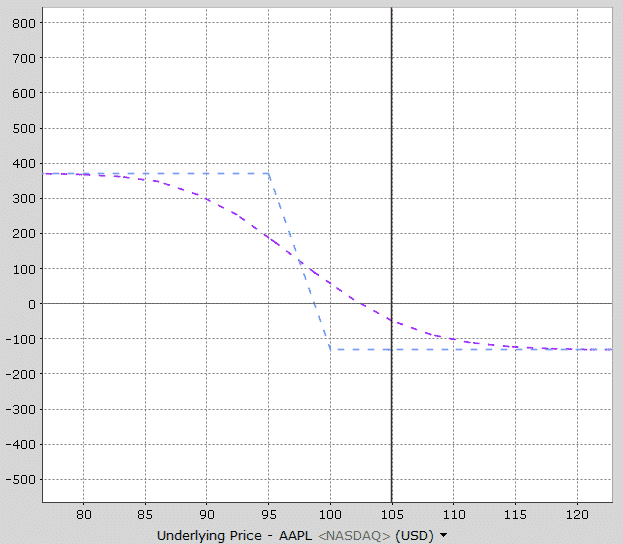See how the purple line is now much closer to the expiration payoff line?

Most brokers will be able to provide this sort of graphical information for you.

I also like to use Option Net Explorer because they can show multiple interim date lines.

Looking at the payoff graph below, notice that there are three interim lines – T+0, T+10 and T+20. This gives the trader a good appreciation of how the trade will progress over time after 10 days and 20 days.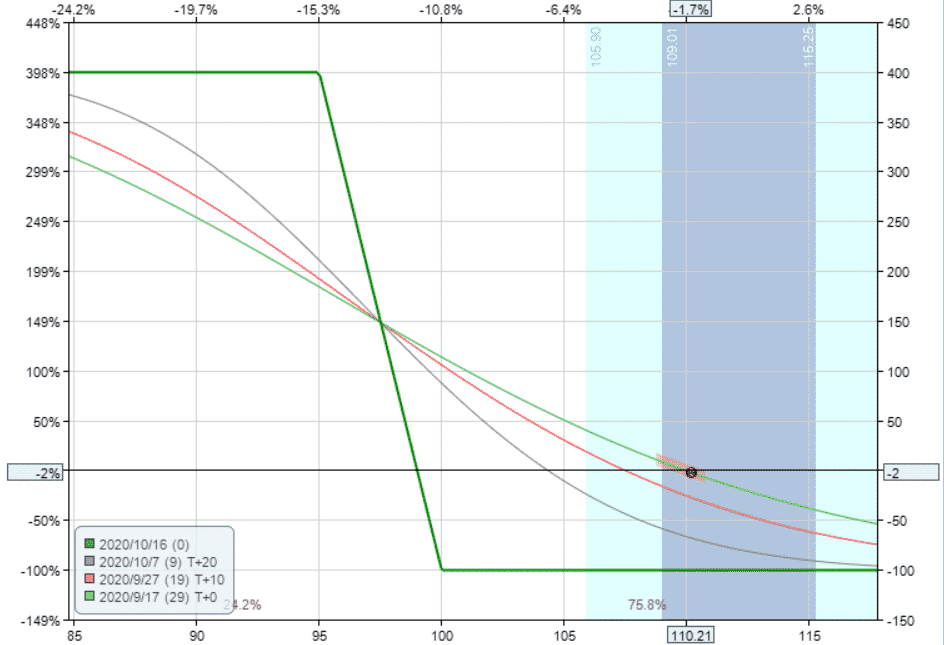## How Changes In Volatility Impact The Payoff Graph

Changes in implied volatility will not have any impact on the payoff graph at expiration, but it will impact the interim dates.

A bear put spread strategy has positive vega, meaning that it will benefit from an increase in implied volatility after placing the trade.

This AAPL trade example has vega of 2.50 which means that for every 1% rise in implied volatility, the trade will make \$2.50 with all other factors being equal.

The opposite is true if implied volatility drops.

Let’s take a look at how a 20% rise in volatility impacts the interim lines.

Notice that the expiration line hasn’t changed at all, but the interim lines have changed. The impact of volatility changes depends on where the stock price is.

Above the mid-point of the spread a rise in volatility helps the trade, but below the mid-point of the spread, volatility hurts the trade.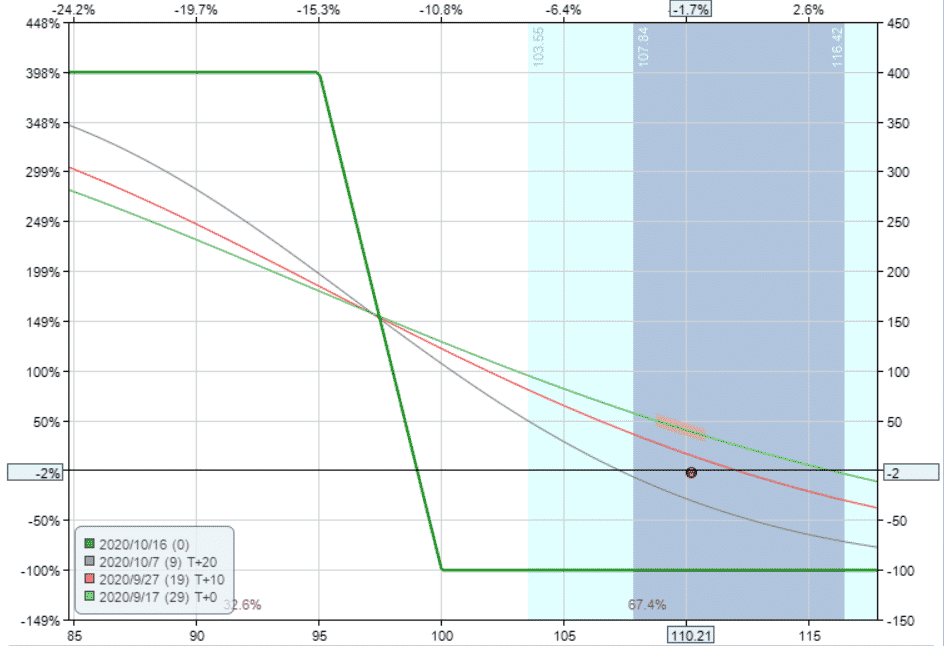The opposite is true if we assume a 20% drop in volatility. Below the mid-point of the spread it helps, above the mid-point it is bad for the trade.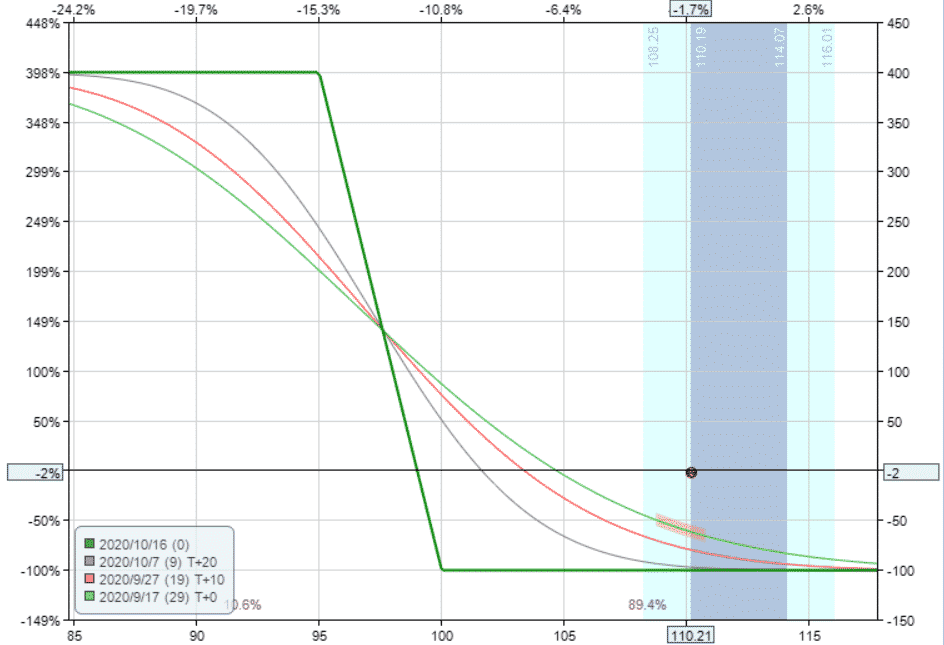With a bear put spread strategy, delta is the main driver of the trade, but we can clearly see that big changes in implied volatility will also affect the trade.

## Bear Put Spread Payoff Diagram Summary

You should now be intimately familiar with the bear put spread option payoff graph. Let’s summarize some key points.

• Bear put spread options are a bearish strategy with limited risk and limited upside
• The maximum loss is equal to the premium paid
• The breakeven price is equal to the long strike price minus the premium paid
• The position will lose value as expiration approaches due to time decay. But, if the stock is below the mid-point of the spread, time decay actually helps the trade.
• As a positive vega trade, increase in implied volatility will help the trade, but only at interim dates and only when the stock is above the mid-point of the spread.

If you’re still having trouble or want to learn more about these concepts, you can check out this 12,000 word beginners guide to options.